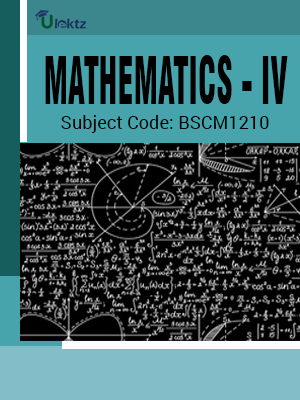uLektz apps

•My WalletMy Order
•My Profile
•My Connections
•My Books
•My Videos
•My Tests
•My Calender
•My Messages
•My Shopping Cart
•My Orders
•Account Settings
•Help

uLektz apps

# Book Details# MATHEMATICS – IV

Course Code:BSCM1210

Author:uLektz

University:

Regulation:2010

Categories:Civil

Format :ePUB3 (DRM Protected)

Type :eBook

FREE

Description :MATHEMATICS – IV of BSCM1210 covers the latest syllabus prescribed by Biju Patnaik University of Technology (BPUT) for regulation 2010. Author: uLektz, Published by uLektz Learning Solutions Private Limited.

Note : No printed book. Only ebook. Access eBook using uLektz apps for Android, iOS and Windows Desktop PC.

##### Topics
###### UNIT-I NUMERICAL METHODS

1.1 Approximation and round of errors, Truncation error and Taylor’s series

1.2 Roots of equation: The bisection method, the false-position method, fixed point iteration, the Newton-Raphson method, Muller’s method

1.3 Linear algebraic equation: LU decomposition, the matrix inverse, Gauss-Seidel method

1.4 Interpolation: Newton divided difference interpolation, Lagrange Interpolation, Newton’s forward and backward interpolation

1.5 Numerical integration: The trapezoidal rule, The Simpson’s rules, Gauss quadrature Ordinary differential equation: Euler’s method,Improvement of Euler’s method, Runge-Kutta methods

###### UNIT-II PROBABILITY

2.1 Probability, Random variables, Probability distributions

2.2 Mean and variance of distribution, Binomial, Poisson and Hyper geometric distributions

2.3 Normal distribution, Distribution of several random variables

###### UNIT-III MATHEMATICAL STATISTICS

3.1 Random sampling, Estimation of Parameters, Confidence Intervals

3.2 Testing of hypothesis, Acceptance sampling, Chi square test for goodness of fit

3.3 Regression Analysis, Fitting Straight Lines, Correlation analysis

### Related Books